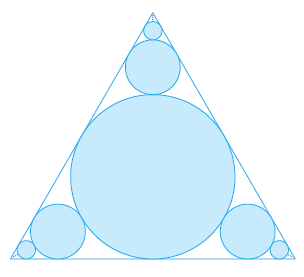# Infinitely Many Circles in an Equilateral TriangleIn the figure there are infinitely many circles approaching the vertices of an equilateral triangle, each circle touching other circles and sides of the triangle. If the triangle has sides of length 1, find the total area occupied by the circles.

I need to find the total area of the circles.

I know this is going to have something to do with summation as a value approaches infinity, but I'm not entirely sure how to approach the problem. Here's what I have so far:

I know that the radius of the central inscribed circle is $$\frac{\sqrt{3}}{6}$$. As such, the area of the first circle is $$A = \pi\left(\frac{\sqrt{3}}{6}\right)^2.$$ Because there are three "branches" of infinite circles, I'm assuming that the answer will look something like: $$A = \pi\left(\frac{\sqrt{3}}{6}\right)^2 + 3 \sum_{1}^{\infty}\text{something}.$$

• Have you learned the formula for the sum of a geometric series? – JimmyK4542 Sep 25 '14 at 1:31
• @JimmyK4542 $\sum_{n=1}^{\infty} a_n = s$? Yes – Alex Sep 25 '14 at 1:36

Since the length of the equilateral triangle is 1, let its median length $x = \frac{\sqrt(3)}{2}$. So, the radius of the first circle is $\frac{x}{3}$. Hence, its area is $A_1 = {\pi}(\frac{x}{3})^2$ The radius of the next three identical circles will be $\frac{x}{9}$. The radius of each progressing circle will be a third of the previous one. So, the sum of the areas of these circles is

$A = A_1+3\sum_{n=1}^{\infty}\frac{\pi x^2}{3^{2n+2}}$ Now, considering the fact that $x=\frac{\sqrt(3)}{2}$,

$3\sum_{n=1}^{\infty}\frac{\pi x^2}{3^{2n+2}} = 3\sum_{n=1}^{\infty}\frac{9 \pi}{4*3^{2n+2}} = \frac{\pi}{4}\sum_{n=1}^{\infty}\frac{1}{9^n}$. Considering the convergence of the geometric with r<1, the total area is given by

$A = A_1 + \frac{\pi}{4} \sum_{n=1}^{\infty} \frac{1}{9^n} = \frac{\pi}{4} + \frac{\pi}{32} = \frac{11 \pi}{96}$. Thus, the total area is $\frac{11 \pi}{96}$

• That's definitely the correct answer, so first and foremost: thanks a lot! I just have one (probably) very simple question: How did you know that the radius of the circle was the median length divided by three? I'm obviously missing some very evident geometric property that's just not coming to mind. – Alex Sep 25 '14 at 3:18
• The circle is tangent to the triangle at all the three sides. So the radius of the circle is perpendicular to the side where the circle is tangent to it. Also, a line through the Center of the circle and the tangent point is orthogonal to the side. Hence it coincides with the median of the triangle since it is also the perpendicular bisector of the equilateral triangle. So, the Center of the circle is at the centroid of the triangle. Thus, the circle's radius is 1/3rd of the median. – dragon7 Sep 25 '14 at 13:34
• I think that the area of $A_1$ should be $\frac{\pi}{12}$ – matt Mar 16 at 15:31

Look at the following figure carefully,As the triangle is equilateral ($$AC$$ is the angle bisector). So, $$\angle ACD = 30^{\circ}$$ $$\tan 30^{\circ} = \frac{AD}{DC} = 2AD\ (\because DC = 1/2)$$ $$\therefore AD = \frac{1}{2\sqrt{3}}$$ This is the radius of the bigger circle, let its area be $$A_1$$ $$\therefore A_1 = \frac{\pi}{12}$$

To calculate the radius of the next smaller circle (let it be $$x$$), please note that

$$AC = \frac{1}{\sqrt{3}}$$

$$AB =\frac{1}{2\sqrt{3}} +x$$

$$\therefore BC = AC - AB =\frac{1}{2\sqrt{3}} -x$$

Note that triangles $$ADC$$ and $$BCE$$ are similar. $$\therefore \frac{AD}{AC} = \frac{BE}{BC}$$

$$\frac{1}{2\sqrt{3}} \times \sqrt{3} = x \times \left( \frac{2\sqrt{3}}{1-2\sqrt{3}x} \right)$$

$$\therefore x = \frac{1}{6\sqrt{3}}$$

Similarly we can find the radii of the next circles. They would be $$\frac{1}{18\sqrt{3}}$$, $$\frac{1}{54\sqrt{3}}, ...$$

The sequence $$\frac{1}{6\sqrt{3}},\frac{1}{18\sqrt{3}}, \frac{1}{54\sqrt{3}}, ...$$ can be generally written as $$\frac{1}{6\sqrt{3}(3)^{n-1}}$$

Total area of these circles,

$$T = \frac{\pi}{12} + 3\sum_{n=1}^{\infty} \pi {\left(\frac{1}{6\sqrt{3}(3)^{n-1}} \right)}^2$$

Notice that, $$\sum_{n=1}^{\infty} \pi {\left(\frac{1}{6\sqrt{3}(3)^{n-1}}\right)}^2 = \sum_{n=1}^{\infty} \pi {\left( \frac{1}{108}\right)}{\left(3^{-(n-1)}\right)}^2$$

=$$\sum_{n=1}^{\infty} \pi {\left( \frac{1}{108}\right)}{\left(3^{-2(n-1)}\right)}$$

=$$\sum_{n=1}^{\infty} \pi {\left( \frac{1}{108}\right)}{\left(\frac{1}{9}\right)}^{n-1}$$

This is a GP with $$a = \frac{\pi}{108}$$ and $$r = \frac{1}{9}$$

For infinite terms, the sum of this GP = $$\frac{a}{1-r} = \frac{\pi}{96}$$

Now, finally,

$$T = \frac{\pi}{12} + 3 \times \frac{\pi}{96} = \frac{11\pi}{96}$$